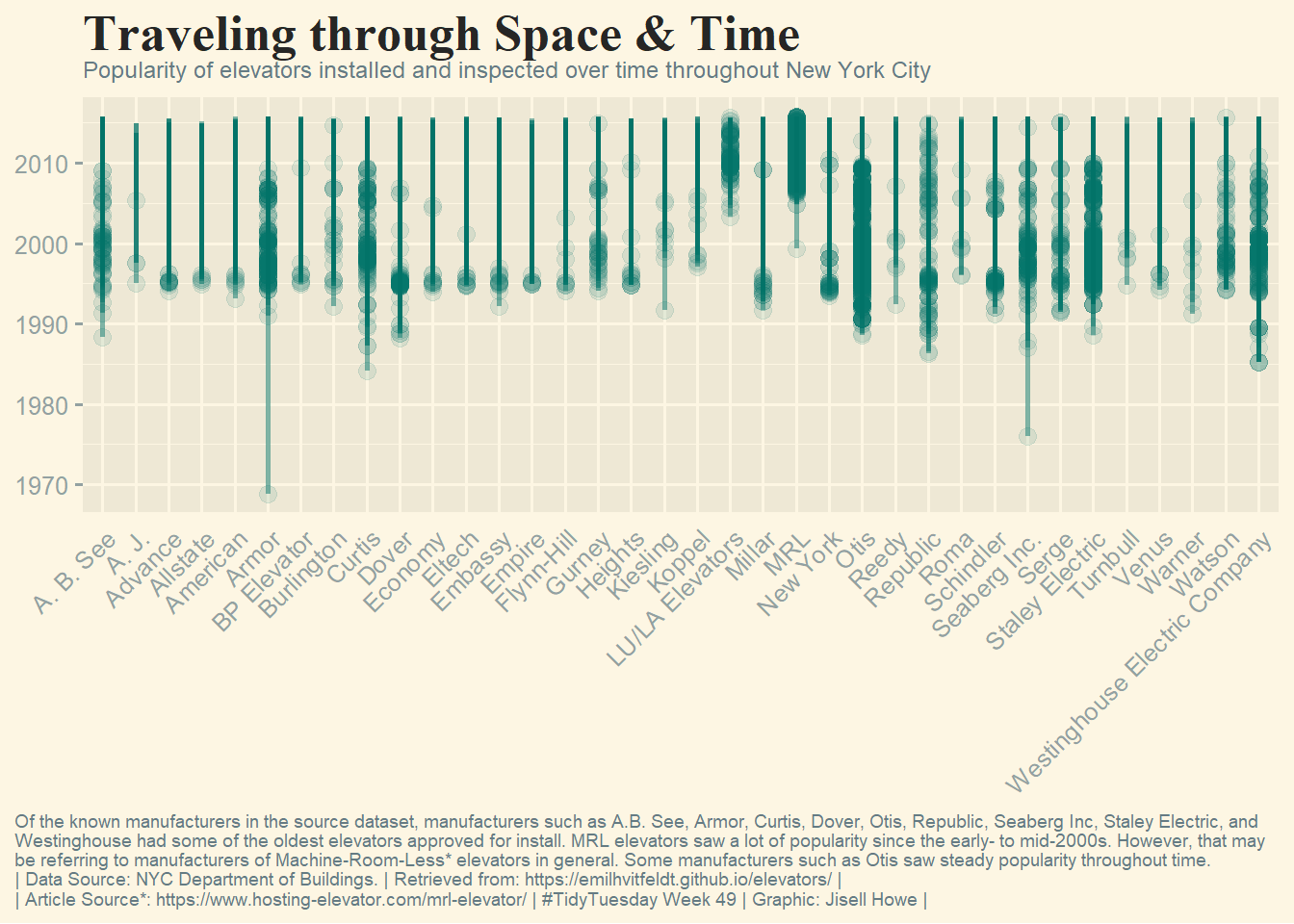# TidyTuesday - 2022W49

tidytuesday
rstats
Author

Jisell Howe, CDT

Published

December 8, 2022

Here is my submission for Tidy Tuesday 2022 W49. Link to code on GitHub.

Show the code
``````remotes::install_github("emilhvitfeldt/elevators")

library(elevators)
library(ggplot2)
library(tidyverse)
library(ggthemes)

#df_mfg <- na.omit(elevators)
df_mfg <- elevators
df_mfg <- filter(df_mfg, manufacturer != "MISSING")
#df_mfg <- filter(na.omit(df_mfg, manufacturer))
#df_mfg <- filter(na.omit(df_mfg, approval_date))
#df_mfg <- filter(df_mfg\$approval_date != NA)
#df_mfg <- filter(na.omit(df_mfg, lastper_insp_date))
df_mfg <- df_mfg[!(is.na(df_mfg\$approval_date)), ]
elevators <- elevators[!(is.na(elevators\$approval_date)), ]
df_mfg\$travel_distance <- as.numeric(df_mfg\$travel_distance)
df_mfg\$bin <- as.numeric(df_mfg\$bin)
df_mfg\$house_number <- as.numeric(df_mfg\$house_number)
df_mfg\$tax_block <- as.numeric(df_mfg\$tax_block)
df_mfg\$zip_code <- as.numeric(df_mfg\$zip_code)
df_mfg <- df_mfg[order(df_mfg\$manufacturer),]

df_mfg_test <- filter(df_mfg, manufacturer == "MRL")
df <- elevators[order(elevators\$approval_date),]

# create plot
palette <- "#027368"
p <- ggplot(df_mfg, aes(x = manufacturer, y = approval_date)) +
theme_solarized_2() +
geom_segment(aes(
x = manufacturer,
y = approval_date,
xend = manufacturer,
yend = lastper_insp_date), size = 1, alpha = 0.5, show.legend = FALSE, color = palette) +
geom_point(size =3, alpha = 0.1, color = palette) +
labs(title = "Traveling through Space & Time",
subtitle = "Popularity of elevators installed and inspected over time throughout New York City",
caption = "Of the known manufacturers in the source dataset, manufacturers such as A.B. See, Armor, Curtis, Dover, Otis, Republic, Seaberg Inc, Staley Electric, and\nWestinghouse had some of the oldest elevators approved for install. MRL elevators saw a lot of popularity since the early- to mid-2000s. However, that may\nbe referring to manufacturers of Machine-Room-Less* elevators in general. Some manufacturers such as Otis saw steady popularity throughout time.\n| Data Source: NYC Department of Buildings. | Retrieved from: https://emilhvitfeldt.github.io/elevators/ |\n| Article Source*: https://www.hosting-elevator.com/mrl-elevator/ | #TidyTuesday Week 49 | Graphic: Jisell Howe | ") +
theme(axis.ticks.x = element_blank(),
axis.text.x = element_text(angle = 45, hjust = 1),
axis.title.x = element_blank(),
axis.title.y = element_blank(),
legend.position="none") +
theme(plot.title = element_text(family = "serif",
face = "bold",
color = "#262625",
size = 20,
hjust = 0,
vjust = 1,
lineheight = 1,
margin = margin(0, 0, 0, 0)),
plot.subtitle = element_text(hjust = 0, size = 9),
plot.caption = element_text(hjust = 0, size = 7),
plot.caption.position = "plot")
p``````Thomas Mock (2022). Tidy Tuesday: A weekly data project aimed at the R ecosystem. https://github.com/rfordatascience/tidytuesday.